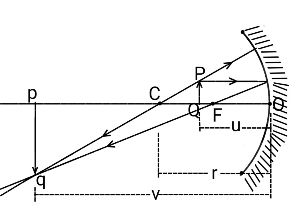# A 1.80-m-tall person stands 9.00 m in front of a large, concave spherical mirror having a radius...

## Question:

A 1.80-m-tall person stands 9.00 m in front of a large, concave spherical mirror having a radius of curvature of 5.00 m. Determine:

(a) The mirror s focal length,

(b) The image distance,

(c) The magnification.

(d) Is the image real or virtual?

(e) Is the image upright or inverted?

## CONCAVE MIRROR FORMULA:Let the radius of curvature of a concave mirror be {eq}r {/eq}.

Therefore the focal length of the mirror will be given by:

{eq}\displaystyle{OF = -f = -\dfrac r2} {/eq}.

The object distance, {eq}OP = -u {/eq}.

The image distance, {eq}Op = -v {/eq}.

The distance of everything that is to the left of the mirror is negative.

In the diagram, a real image has been formed by the mirror-reflected rays actually meeting.

{eq}PQ {/eq} is the object and {eq}pq {/eq} is its real inverted image.

The magnification {eq}(m) {/eq} by the mirror is defined as the ratio of the height of the image {eq}(pq) {/eq} to that of the object {eq}(PQ) {/eq} and can be proved to be:

{eq}\displaystyle{m = \dfrac{pq}{PQ} = -\dfrac vu} {/eq}.

{eq}u {/eq} is always negative.

If {eq}v {/eq} is negative, {eq}m {/eq} is also negative and the image will be real and inverted.

If {eq}v {/eq} is positive, {eq}m {/eq} will be negative and the image will be virtual and upright.

If {eq}|m| > 1 {/eq}, the image will be enlarged and {eq}|m| < 1 {/eq}, the image will be dwarfed.

The mirror (whether concave mirror or convex mirror) formula is given as:

{eq}\displaystyle{\dfrac 1v + \dfrac 1u = \dfrac 1f = \dfrac 2r} {/eq}.

The distance of the image from the mirror can be given by:

{eq}\displaystyle{v = \dfrac{ru}{2u - r}} {/eq}.

The magnification of the image is given by:

{eq}\displaystyle{\begin{align*} m &= -\dfrac{v}{u}\\ m &= -\dfrac{\dfrac{ru}{2u - r}}{u}\\ m &= -\dfrac{r}{2u - r}\\ \end{align*}} {/eq}

GIVEN

• The radius of curvature of the concave mirror: {eq}r = -5.00 \ \rm m \ \ \ \ \ \ \ \ (\text{since it is concave mirror}) {/eq}.
• The distance of the man from the concave mirror: {eq}u = -9.00 \ \rm m \ \ \ \ \ \ \ \ \ (\text{since object distance is always considered negative}) {/eq}.

(a) The focal length of the mirror is given by:

{eq}\displaystyle{\begin{align*} f &= \dfrac{r}{2}\\ f &= \dfrac{-5.00 \ \rm m}{2}\\ f &= \boxed{{-2.50 \ \rm m}}\\ \end{align*}} {/eq}

(b) The distance of the image will be given by:

{eq}\displaystyle{\begin{align*} v &= \dfrac{ru}{2u - r}\\ v &= \dfrac{(-5.00 \ \rm m)\times (-9.00 \ \rm m)}{2\times (-9.00 \ \rm m) - (-5.00 \ \rm m)}\\ v &\approx \boxed{{-3.46}}\\ \end{align*}} {/eq}

The negative sign indicates the image will be formed on the side of the object.

(c) The magnification of the image will be given by:

{eq}\displaystyle{\begin{align*} m &= -\dfrac{r}{2u - r}\\ m &= -\dfrac{(-5.00 \ \rm m)}{2\times (-9.00 \ \rm m) - (-5.00 \ \rm)}\\ m &\approx \boxed{{-0.385}}\\ \end{align*}} {/eq}

(d) The value of {eq}m {/eq} is negative.

Hence, the image will be a real one.

(e) As {eq}m {/eq} is negative, the image will be inverted.